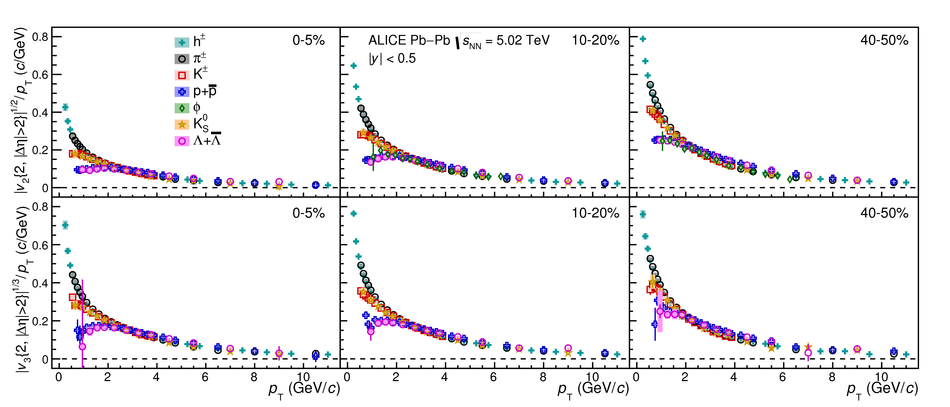Figure 18

 Centrality dependence of $|v_{\rm n}|^{1/{\rm n}} / \pt{}$ of inclusive charged particles , \pipm{}, \kapm{}, p+\pbar{}, \lambdas{}, \kanull{}, and the $\phi$-meson for $n=2$ (upper panels) and $n=3$ (lower panels). Statistical and systematic uncertainties are shown as bars and boxes, respectively.